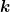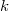﻿

### On solutions of fractional kinetic equations involving the generalized$\boldsymbol{k}$-Bessel function

#### Abstract

In the paper, the authors obtain solutions of fractional kinetic equations involving the generalized$k$-Bessel function given by Mondal. These results are general in nature and are useful to investigate many problems in applied sciences.

DOI Code: 10.1285/i15900932v37n2p11

Keywords: solution; fractional kinetic equation; Laplace transform; \$k\$-Bessel function

Full Text: PDF